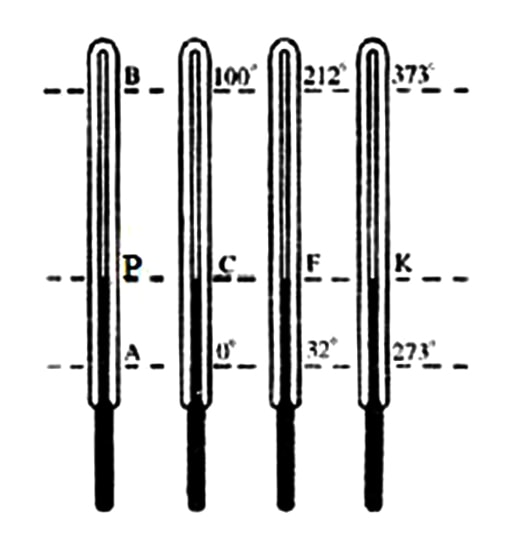Physics

# Explain Relation among Celsius, Fahrenheit and Kelvin scale

A scale of temperature is essential to indicate the temperature of a body accurately. Two definite temperatures are taken as constant to prepare a temperature scale. These two temperatures are called fixed points. The two fixed points are lower fixed point and the upper fixed point. At standard pressure and at the temperature at which pure ice melts to water or pure water freezes to ice is called the lower fixed point.

It is also termed as freezing point or ice point. Again at standard pressure and at the temperature at which pure boiling water converts into water vapor is called upper fixed point. The upper fixed point is also called boiling point or steam point.The temperature difference between the two fixed points is called the fundamental interval of a thermometer. The fundamental interval i.e. the space in between the two fixed points is divided in different ways to prepare different scales of temperature.

There are three scales of temperature in use — Celsius, Fahrenheit and Kelvin scale. The units of temperature of these three scales are °C, °F and K respectively. The lower fixed point in Celsius scale is 0°C, in Fahrenheit scale 32°F and in Kelvin scale it is 273K. The upper fixed point in Celsius scale is 100°C, in Fahrenheit scale 212°F and in Kelvin scale it is 373K.